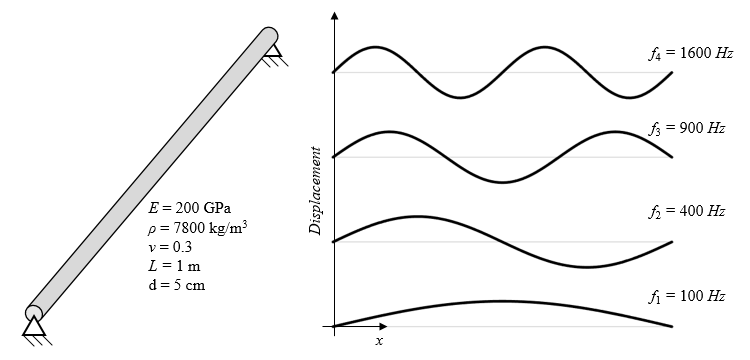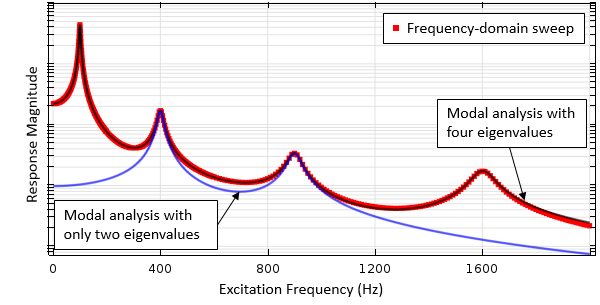# 第 1 部分：模拟线性系统的谐波激励

2016年 8月 10日

### 工程和科学中的时谐载荷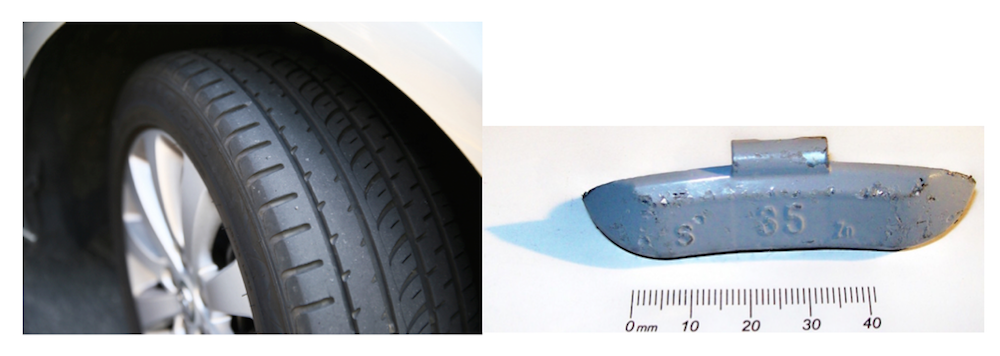(1)

\begin{align}
M u_{tt} + C u_t + \nabla \cdot (-K \nabla u) = F &\text { on } \Omega \\
\mathbf{n} \cdot (K \nabla u) + Au = f &\text{ on } \Gamma_1 \\
u = g &\text{ on } \Gamma_2
\end{align}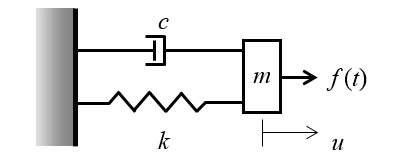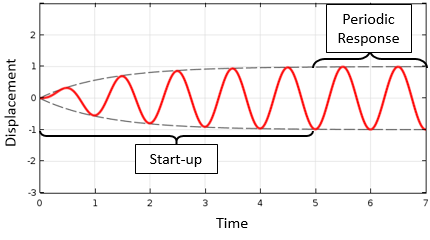u(\mathbf{x},t)=\tilde u(\mathbf{x}) \exp(j \omega t)

(2)

\begin{align}
-\omega^2 M \tilde u + j \omega C \tilde u+ \nabla \cdot (-K \nabla \tilde u) = \tilde F & \text { on } \Omega \\
\mathbf{n} \cdot (K \nabla \tilde u) + A \tilde u = \tilde f &\text{ on } \Gamma_1 \\
\tilde u = \tilde g &\text{ on } \Gamma_2
\end{align}

### 分析频域中的问题

COMSOL Multiphysics® 软件包含一套不同的算法，适用于求解上述方程（等式(2))。最直接的方法是频域 求解器。该求解器将单个频率或离散频率范围作为输入进行仿真。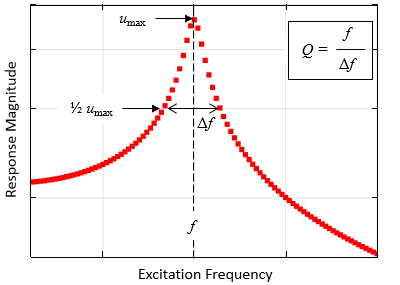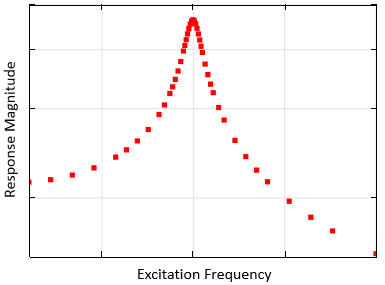AWE 求解器在响应随频率显著变化的范围内引入了额外的计算点。

(3)

\begin{align}
-\omega^2 M \tilde u + j \omega C \tilde u+ \nabla \cdot (-K \nabla \tilde u) = 0 & \text { on } \Omega \\
\mathbf{n} \cdot (K \nabla \tilde u) + A \tilde u = 0 &\text{ on } \Gamma_1 \\
\tilde u = 0 &\text{ on } \Gamma_2
\end{align}